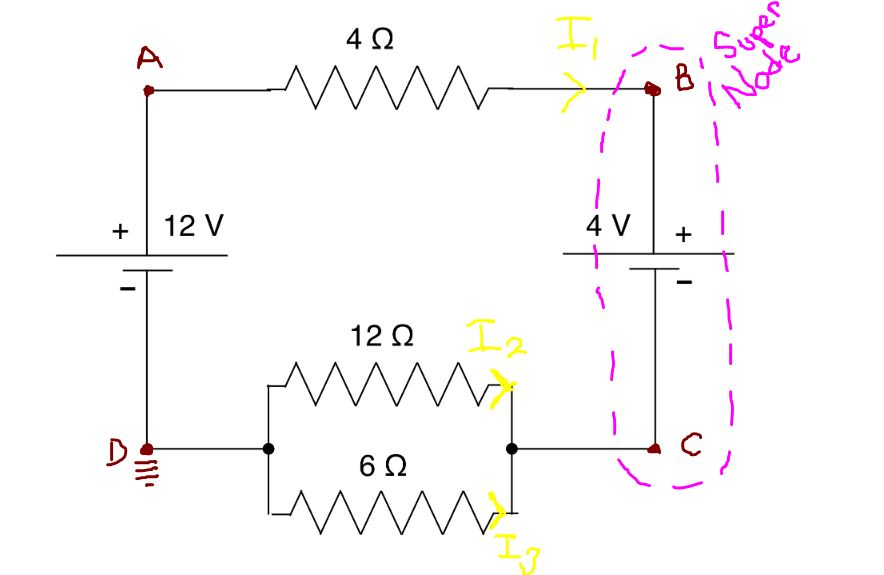1AVD = 0V

VA = 12 V

VB – VC = 4V

apply KCL at node B-C, assume all currents into node

I1 + I2 + I3 = 0

I1(12-B)/4
I2 = (0-C)/12
I3 = (0-C)/6

I1 + I2 + I3 = 0

(12-B)/4   +  (0-C)/12 +  (0-C)/6 = 0

B + C = 12   –> VB + VC = 12V

VB – VC = 4V

VB + VC = 12V

2VB = 16V

VB = 8V

VC = 4V

I1(12-B)/4 = (12V-VB)/4Ω = (12V-8V)/4Ω = (4V)/4Ω = 1A
I2 = (0-C)/12 = (-VC)/12Ω = (-4V)/12Ω =  -1/3 A
I3 = (0-C)/6= (-VC)/6Ω = (-4V)/6Ω =  -2/3 A

1B

P = IV3      V = IR

∴P = I·(IR) = I2R

P = (IA)2·(4Ω) = 4W = 4 J/s

E = PΔt = 4 J/s · 20s = 80J    (this equation is from the conservation of energy law)

1C

P = IV = I1 · (4V)  = IA · (4V) = 4W

(Note:  the power is absorbed as the current is flowing from +Ve → -Ve

2A

T = RC : [ V/A · C/V = C/A ]

T = (10×10<sup>3</sup> Ω) · (100 x 10<sup>-6</sup> F) = 1000 x 10<sup>-3</sup> ΩF

Ω = V/A    F = (A·S)/V     Ω·F = V/A  · (A·S)/V  = S

T=1s

2B

I(t) = I<sub>0</sub>(e<sup>-t/2</sup>)

Ohms law:  I<sub>0</sub> = V/R = 20/(10×10<sup>6</sup>) = 2 x 10<sup>-6</sup> A = 2mA

“find (t) when I(t) = .5I<sub>0</sub>”

I(t) = .5I<sub>0</sub> = I<sub>0</sub>(e<sup>-t/x</sup>) → .5 = e<sup>(-t/x)</sup>

ln.5 = lne<sup>(-t/x)</sup> =ln.5 = -t/x

Tln.5 = t = 1(ln.5) = ln·5s

t = -(ln·5)s